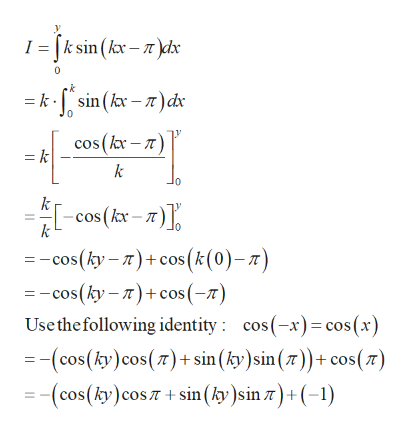# Evaluate ∫y0ksin(kx−π)dx where y and k are independent of x, and y is given by the solution to the equation cos(ky)=0.57 .

Question
2 views

Evaluate ∫y0ksin(kx−π)dx where y and k are independent of x, and y is given by the solution to the equation cos(ky)=0.57 .

check_circle

Step 1

Given:

Step 2

To evaluate the ...help_outlineImage TranscriptioncloseI = [ksin (kx-)dx = ksin(kx-)dx cos (kx-) = k k cos (k- = -cos(ky-)cos(k(0)-z) =-cos(ky-)cos (-) Use the following identity cos(-x)cos (x) (cos(ky) cos(7)+ sin (ky)sin ()+ cos() (cos(ky) cos +sin (ky )sin z) (-1) fullscreen

### Want to see the full answer?

See Solution

#### Want to see this answer and more?

Solutions are written by subject experts who are available 24/7. Questions are typically answered within 1 hour.*

See Solution
*Response times may vary by subject and question.
Tagged in

### Integration